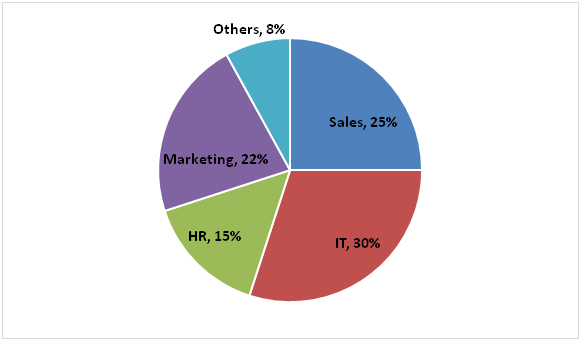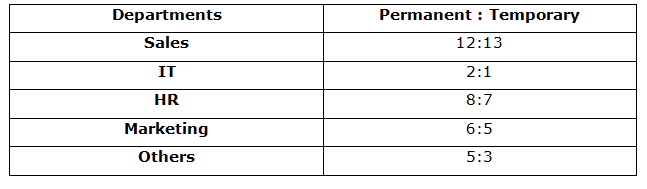# NIACL AO Mains– Quantitative Aptitude Questions Day- 13

Dear Readers, Bank Exam Race for the Year 2019 is already started, To enrich your preparation here we have providing new series of Practice Questions on Quantitative Aptitude – Section. Candidates those who are preparing for NIACL AO Mains 2019 Exams can practice these questions daily and make your preparation effective.

[WpProQuiz 5190]

Directions (Q. 1 – 5): What value should come in place of (?) in the following questions?

1) (2156 + 534 + 302 + 158) ÷ (21 + 35 – ? + 26) = 42

a) 12

b) 19

c) 7

d) 23

e) None of these

2) (4/7) of ? – (2/9) of (81/40) of 3600 = 18 % of 2200

a) 3528

b) 3056

c) 2892

d) 2764

e) None of these

3) (3/11) ÷ (72/341) × 288 – 440 + 56 % of 1500 = ?

a) 656

b) 598

c) 814

d) 772

e) None of these

4) 40 % of 700 + 55 % of 800 – 32 % of 750 = ?

a) 390

b) 480

c) 520

d) 560

e) None of these

5) 3 (5/9) + 6 (1/3) – 5 (1/18) + 11 (1/12) = ? + 4 (1/9)

a) 10 (5/6)

b) 12 (4/9)

c) 11 (29/36)

d) 9 (11/12)

e) None of these

Directions (6 – 10): Study the following information carefully and answer the questions given below:

The following pie chart represents percentage wise distribution of number of employees working in different departments of an organisation in the year 2017.

Total number of employees in the organisation in 2017 = 30000The following table represents respective ratio of number of permanent and temporary employees among them.6) Out of the number of permanent employees in the Sales department in 2017, respective ratio of males and females is 11:7 and out of the number of temporary employees in the Sales department in 2017, respective ratio of males and females is 7:6. Find the respective ratio of total number of males and total number of females in the Sales department of the organisation in 2017.

a) 35:31

b) 43:47

c) 43:32

d) 49:43

e) None of these

7) Total number of employees in the organisation in 2018 is increased by 20% from the previous year and number of employees in the IT department of the organisation in 2018 is increased by 5% from the previous year. Number of employees in the IT department in 2018 is what percent of the total number of employees in the organisation in 2018?

a) 22.20%

b) 25.12%

c) 24.45%

d) 26.25%

e) None of these

8) Find the difference between total number of permanent employees in Sales department and HR department together in 2017 and total number of permanent employees in IT department and Marketing department together in 2017.

a) 3600

b) 4800

c) 2400

d) 3000

e) None of these

9) Number of temporary employees in which department of the organisation is maximum in 2017?

a) Sales

b) IT

c) HR

d) Marketing

e) Others

10) Number of temporary employees in IT department of the organisation in 2017 is what percent of the number of permanent employees in other departments of the organisation in that year?

a) 250%

b) 200%

c) 100%

d) 150%

e) None of these

Direction (1-5) :

(2156 + 534 + 302 + 158) ÷ (21 + 35 – x + 26) = 42

3150/(82 – x) = 42

3150/42 = 82 – x

75 = 82 – x

X = 82 – 75 = 7

(4/7) of x – (2/9)*(81/40)*3600 = (18/100)*2200

(4/7)*x – 1620 = 396

(4/7)*x = 396 + 1620 = 2016

X = 2016*(7/4) = 3528

(3/11)*(341/72)*288 – 440 + (56/100)*1500 = x

X = 372 – 440 + 840 = 772

(40/100)*700 + (55/100)*800 – (32/100)*750 = x

X = 280 + 440 – 240 = 480

3 (5/9) + 6 (1/3) – 5 (1/18) + 11 (1/12) – 4 (1/9) = x

X = (3 + 6 – 5 + 11 – 4) (5/9 + 1/3 – 1/18 + 1/12 – 1/9)

X = 11 [(20 + 12 – 2 + 3 – 4)/36] = 11 (29/36)

Direction (6-10) :

Total number of employees in the Sales department in 2017 = 25/100 x 30000 = 7500

Total number of permanent employees in the Sales department in 2017

= 12/25 x 7500 = 3600

Number of male permanent employees in the Sales department in 2017

= 11/18 x 3600 = 2200

Number of female permanent employees in the Sales department in 2017

= 7/18 x 3600 = 1400

Total number of temporary employees in the Sales department in 2017

= 13/25 x 7500 = 3900

Number of male temporary employees in the Sales department in 2017

= 7/13 x 3900 = 2100

Number of female temporary employees in the Sales department in 2017

= 6/13 x 3900 = 1800

Required ratio = (2200 + 2100): (1400 + 1800)

= 4300: 3200

= 43:32

Total number of employees in the organisation in 2018 = 30000 x 120/100

= 36000

Number of employees in the IT department of the organisation in 2017

= 30/100 x 30000 = 9000

Number of employees in the IT department of the organisation in 2018

= 9000 x 105/100 = 9450

Required percentage = 9450/36000 x 100 = 26.25%

Number of permanent employees in Sales department

= 12/25 x 25/100 x 30000 = 3600

Number of permanent employees in HR department = 8/15 x 15/100 x 30000

= 2400

Number of permanent employees in IT department = 2/3 x 30/100 x 30000

= 6000

Number of permanent employees in Marketing department

= 6/11 x 22/100 x 30000

= 3600

Required difference = (6000 + 3600) – (3600 + 2400)

= 9600 – 6000

= 3600

Number of temporary employees in Sales department in 2017

= 13/25 x 25/100 x 30000 = 3900(Maximum)

Number of temporary employees in IT department in 2017

= 1/3 x 30/100 x 30000 = 3000

Number of temporary employees in HR department in 2017

= 7/15 x 15/100 x 30000 = 2100

Number of temporary employees in Marketing department in 2017

= 5/11 x 22/100 x 30000 = 3000

Number of temporary employees in other departments in 2017

= 3/8 x 8/100 x 30000 = 900

Number of temporary employees in IT department in 2017

= 1/3 x 30/100 x 30000 = 3000

Number of permanent employees in other departments in 2017

= 5/8 x 8/100 x 30000 = 1500

Required percentage = 3000/1500 x 100 = 200%